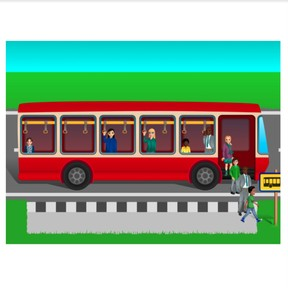Subtraction to 10 via bus example

# Subtraction to 10 via bus example

No account needed.8,000 schools use Gynzy92,000 teachers use Gynzy1,600,000 students use Gynzy

## General

Students learn to subtract numbers to 10 using the bus method.

1.OA.A

## Relevance

If students want to be able to subtract well, they need to be able to subtract numbers to 10. Using the bus method helps students understand the subtraction process by placing it in a visual story form.

## Introduction

Start by showing a picture of a bus to the class. Ask who in class has ever been on a bus. Ask where they went, and if it was busy in the bus. Then have students practice counting quantities by having students count the number of students who are waiting for the bus.

## Development

Discuss that it is important to be able to calculate subtraction problems to 10 using the bus ride as context. Using the three images, explain how bus problems work. The first image shows how many passengers are on the bus as it arrives. The second shows how many passengers exit the bus at the stop. The third image shows how many passengers are left on the bus as it leaves. You can determine this third image total by taking away the number of exiting passengers from the total number of passengers from the first image. Show the students the subtraction problem that matches the images, namely 2 - 1 = 1. Ask students to name which number is the first number of the subtraction problem (minuend) which is the second number of the subtraction problem (subtrahend) and what is the total, or difference. Tell students that they can check their work by adding their total (difference) and second number (subtrahend) together.
Next practice two more bus examples on the interactive whiteboard. Explain to students that the images will now stay the same- you can have all the information in just one image. Practice this with two bus examples and show students that they can also solve using the bus method even when they can't count the individual passengers, but are given numbers to represent the number of passengers on the bus. Practice with the students.

To check that students are able to subtract numbers to 10 using the bus method, you can ask the following questions:
- Which steps do you take in solving subtraction on the bus?
- How many passengers are still on the bus if there are 3 passengers and 2 exit the bus?
- How many passengers are still on the bus if there are 8 passengers and 5 exit the bus?

## Guided practice

Students are first asked to determine totals or subtraction problems that match the bus examples given with countable passengers. Some of the questions are multiple choice. Then students are asked to solve problems with only numbers, an no countable passengers. Students are finally asked to solve an entire bus example with only numbers.

## Closing

Repeat the learning goal and walk through the steps needed to solve a subtraction problem using the bus method. Ask students to solve a problem in which a bus has 9 passengers and 7 exit the bus. Ask students what the subtraction problem looks like. Next play the game "blind bus driver". In this game- select one student to be the bus driver and select a few students to be passengers on their bus. The bus driver closes their eyes and a few students sneak "off" the bus. The bus driver (with eyes still closed) must guess how many students are left in the bus and say what that subtraction problem is.

## Teaching tips

Students who have difficulty can be helped by repetition of the steps required to solve subtraction problems using the bus method. First count the total number of passengers in the bus, then count the total number of passengers exiting the bus, and then counting the total number of passengers left in the bus. Students can also use blocks or other manipulatives to represent the passengers on the bus, and have them remove blocks as the passengers exit the bus. Start with smaller subtraction problems (for example to 5) to build confidence before expanding to 10.

## Instruction materials

blocks

### The online teaching platform for interactive whiteboards and displays in schools

• Save time building lessons

• Manage the classroom more efficiently

• Increase student engagement